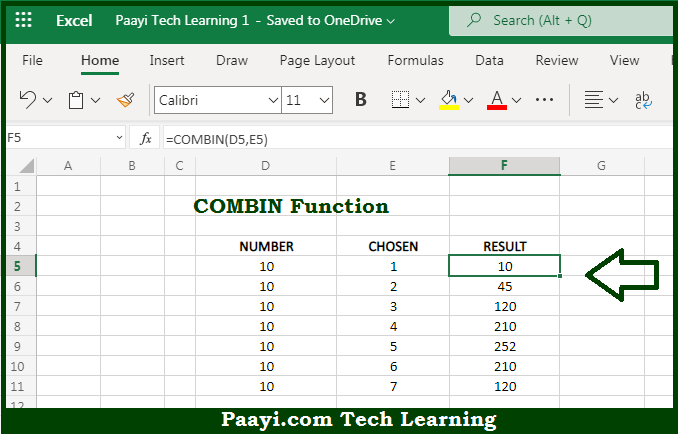# Learn How to Use Microsoft Excel COMBIN Function

Written by | 0 Comments | 527 Views

In this article, you will learn how to use the Microsoft Excel COMBIN function and its prime function in Microsoft Excel. You will also get to know the Microsoft Excel COMBIN function return value and syntax with the help of some examples.

Microsoft Excel COMBIN Function

The main purpose of the Microsoft Excel COMBIN function is to get the number of combinations without repetitions. That implies, with the help of the COMBIN function you can able to return the number of combinations for the given number of the items. It should be noted that the COMBIN function does not allow repetitions. To count combinations that allow repetitions, use the COMBINA function. Also, the COMBIN returns a #VALUE! error value if either argument is not numeric. If the number is less than the number chosen, COMBIN returns #NUM! So, with the help of the COMBIN function, you can able to get the binomial distribution of the probability.

Return Value of COMBIN Function

The return value will be the number of combinations as an integer.

Syntax of COMBIN Function

=COMBIN(number, number-selected)

Where the arguments:

• number: This is the total number of items.
• number-selected: This is the number of items in each combination.

## How to Use Microsoft Excel COMBIN Function?So we know that Microsoft Excel COMBIN function you can able to get the number of combinations without repetitions. That implies, with the help of the COMBIN function you can able to return the number of combinations for the given number of items. It should be noted that the COMBIN function does not allow repetitions. To count combinations that allow repetitions, use the COMBINA function. Also, the COMBIN returns a #VALUE! error value if either argument is not numeric. If the number is less than the number chosen, COMBIN returns #NUM! So, with the help of the COMBIN function, you can able to get the binomial distribution of the probability.